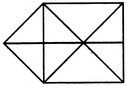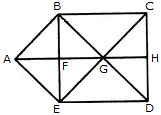# Non Verbal Reasoning - Analytical Reasoning - Discussion

Discussion Forum : Analytical Reasoning - Section 1 (Q.No. 18)
18.

Find the number of triangles in the given figure.15
16
17
18
Explanation:

The figure may be labelled as shown.The simplest triangles are ABF, BFG, BCG, CGH, GHD, GED, EFG and AFE i.e. 8 in number.

The triangles composed of two components each are ABG, BGE, AGE, ABE and GCD i.e. 5 in number.

The triangles composed of three components each are BCD, CDE, BED and BCE i.e. 4 in number.

Thus, there are 8 + 5 + 4 = 17 triangles in the figure.

Discussion:
3 comments Page 1 of 1.

Mithila said:   5 years ago
Right. @Kamlesh.

Kamlesh said:   6 years ago